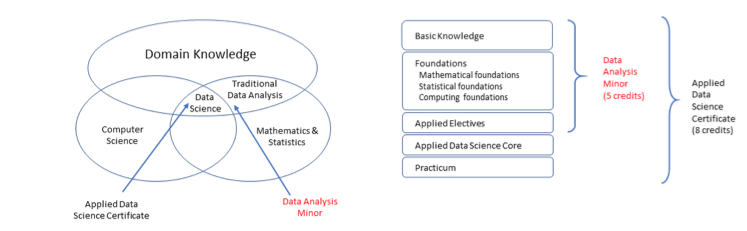# Data Analysis Minor

Department website: http://www.wesleyan.edu/qac/index.html

## Introduction

The Data Analysis Minor provides a basic introduction to data analysis, including the theory and practical skills needed to collect and prepare data for analysis, explore and visualize data, build models and test hypotheses, discover insights, and communicate results in meaningful ways. The Data Analysis Minor is designed to strengthen the student's ability to apply statistical, mathematical, and programming methods to traditional areas of empirical research within their fields.## Minor Requirements

Basic Knowledge Courses
Select one of the following:1
Quantitative Methods for the Biological and Environmental Sciences
Elementary Statistics
Statistics: An Activity-Based Approach
Applied Data Analysis
Applied Data Analysis
Digging the Digital Era: A Data Science Primer
An Introduction to Data Journalism
Mathematical, Statistical, and Computing Foundation Courses
Select two courses from the following, each from a different group:2
Mathematical Foundations
Vectors and Matrices
Linear Algebra
Discrete Mathematics
Graph Theory
Applied Vectors and Matrices
Statistical Foundations
Introductory Econometrics
An Introduction to Probability
Mathematical Statistics
Computing Foundations
Bioinformatics Programming
Introduction to Programming
How to Design Programs
Computer Science I
Computer Science II
Applied Electives
Select two credits from the following:2
Introduction to GIS
Introduction to Forecasting in Economics and Finance
Empirical Methods for Political Science
Computational Physics
Introduction to Survey Design and Analysis
Introduction to (Geo)Spatial Data Analysis and Visualization
Proseminar: Machine Learning Methods for Audio and Video Analysis
Introduction to Network Analysis
Data Visualization: An Introduction
QAC251ZData Visualization: An Introduction1
Exploratory Data Analysis and Pattern Discovery
Experimental Design and Causal Inference
Longitudinal Data Analysis (0.5 credit)
Hierarchical Linear Models (0.5 credit)
Latent Variable Analysis (0.5 credit)
Survival Analysis (0.5 credit)
Applied Time Series Analysis
Bayesian Data Analysis: A Primer (0.5 credit)
Advanced R: Building Open-Source Tools for Data Science
can count QAC 380 or 381, not both
Introduction to Statistical Consulting
QAC Praxis Service Learning Lab
Applications of Machine Learning in Data Analysis
Quantitative Textual Analysis: Introduction to Text Mining
NOTE: at least one of the electives should be a 300 level course